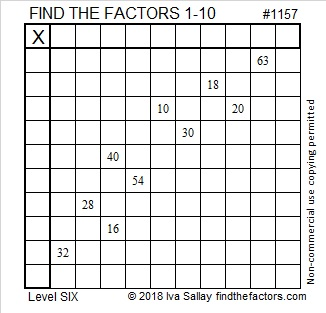# 1157 and Level 6

The more you solve these puzzles, the easier most of them become. This one is no exception. Can you figure out the logic needed to make the first move?Print the puzzles or type the solution in this excel file: 10-factors-1148-1160

Now I’ll share some facts about the number 1157:

• 1157 is a composite number.
• Prime factorization: 1157 = 13 × 89
• The exponents in the prime factorization are 1 and 1. Adding one to each and multiplying we get (1 + 1)(1 + 1) = 2 × 2 = 4. Therefore 1157 has exactly 4 factors.
• Factors of 1157: 1, 13, 89, 1157
• Factor pairs: 1157 = 1 × 1157 or 13 × 89
• 1157 has no square factors that allow its square root to be simplified. √1157 ≈ 34.014734² + 1² = 1157
31² + 14² = 1157

1157 is the hypotenuse of FOUR Pythagorean triples:
68-1155-1157 calculated from 2(34)(1), 34² – 1², 34² + 1²
445-1068-1157 which is (5-12-13) times 89
507-1040-1157 which is 13 times (39-80-89)
765-868-1157 calculated from 31² – 14², 2(31)(14), 31² + 14²

1157 is a palindrome in two different bases:
It’s 2D2 in BASE 21 (D is 13 base 10) because 2(21²) + 13(21) + 2(1) = 1157
101 in BASE 34 because 34² + 1 = 1157

This site uses Akismet to reduce spam. Learn how your comment data is processed.